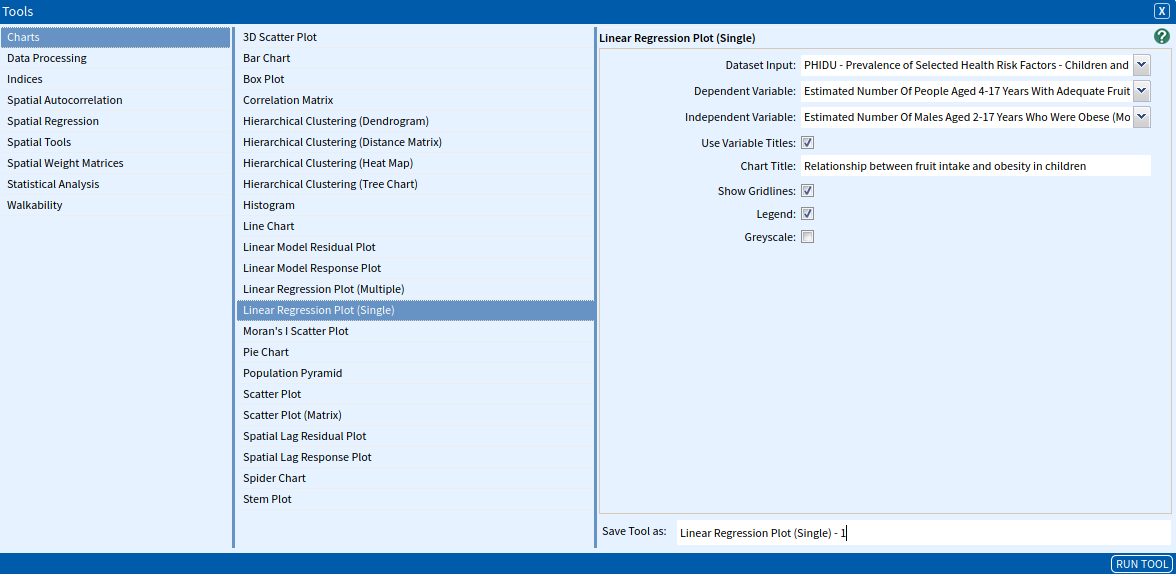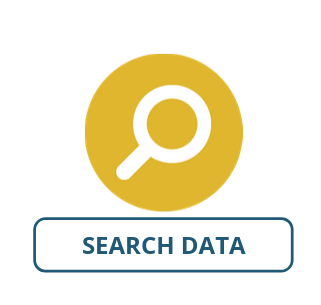# Linear Regression Plot (Single)

The Linear Regression Plot (Single) tool allows you to produce a chart displaying a simple linear regression. A linear regression plot allows you to visualise your variables with more information than that of the scatterplot. The linear regression plot shows you the mathematical relationship between the variables. It is important to use the linear regression plot when you have an idea about causation between your variables, rather than just a correlation.

Simple linear regression plot is a scatter plot with a regression line that shows the general direction (trend) that a group of points (plots) seem to be heading. The plots show the relationship between a scalar dependent variable Y and an explanatory variable denoted X.

### SET UP

To illustrate the Linear Regression Plot (Single) tool, we will run it on a dataset within Tasmania to visualise the connection between fruit intake and obesity in children. Prepare the context by:

• Select Tasmania as your area.
• Select PHIDU – Prevalence of Selected Health Risk Factors – Children and Youth (LGA) 2014-2015 as your dataset with the following attributes:
• LGA Code 2016
• LGA Name 2016
• Estimated Number Of People Aged 4-17 Years With Adequate Fruit Intake (Modelled Estimates) 2014-15 ASR per 100
• Estimated Number Of Males Aged 2-17 Years Who Were Obese (Modelled Estimates)* 2014-15 ASR per 100

Once you have added the dataset and the selected attributes, you are ready to use the Linear Regression Plot (Single) tool – follow on to learn about the input options.

### Inputs

Once you have set up your data, open the Linear Regression Plot (Single) tool (Tools → Charts → Linear Regression Plot (Single)). The input fields are as follows:

• Dataset Input: The dataset containing the variables that you would like to run through the tool. Select: PHIDU – Prevalence of Selected Health Risk Factors – Children and Youth (LGA) 2014-2015
• Dependent Variable: This is where we select the variable that we want to model or regress on the independent variable. Select: Estimated Number Of People Aged 4-17 Years With Adequate Fruit Intake (Modelled Estimates) 2014-15 ASR per 100
• Independent Variable: This is where we select the variable that we want to test as the predictor for the dependent variable. Select: Estimated Number Of Males Aged 2-17 Years Who Were Obese (Modelled Estimates)* 2014-15 ASR per 100
• Use Variable Titles: Check this box to have “human-readable names” on your output chart. For this tutorial, we can name it Relationship between fruit intake and obesity in children.
• Chart Title: This is where we enter the name that we want to give the resultant plot.
• Show Gridlines: Check this box if you want gridlines for your graph.
• Legend: Check this box if you want to include a legend for your graph.
• Greyscale: Check this box if you want your graph to be produced in greyscale.

A summary of the inputs to explore our case can be viewed in the image below, once complete click Run Tool.### Outputs

Once you have run the tool, click the Display button which appears in the pop-up dialogue box. This should open up a chart tool looking like the one shown below.The output provides the estimated linear regression linear equation and indicates a negative relationship between adequate fruit intake in children and childhood obesity.

### Looking for Spatial Data?

You can browse the AURIN Data Discovery:### How can you Create Impact?